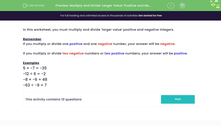# Multiply and Divide 'Larger Value' Positive and Negative Integers

In this worksheet, students carry out multiplication and division of larger value positive and negative integers.Key stage:  KS 3

Curriculum topic:   Number

Curriculum subtopic:   Use Four Operations for All Numbers

Difficulty level:#### Worksheet Overview

In this worksheet, you must multiply and divide 'larger value' positive and negative integers.

Remember

If you multiply or divide one positive and one negative number, your answer will be negative.

If you multiply or divide two negative numbers or two positive numbers, your answer will be positive.

Examples

5 × -7 = -35

-12 ÷ 6 = -2

-8 × -6 = 48

-63 ÷ -9 = 7

### What is EdPlace?

We're your National Curriculum aligned online education content provider helping each child succeed in English, maths and science from year 1 to GCSE. With an EdPlace account you’ll be able to track and measure progress, helping each child achieve their best. We build confidence and attainment by personalising each child’s learning at a level that suits them.

Get started